# How to Remove Leading Zeros in Excel (3 Easy Ways)

If you’re dealing with a spreadsheet that was not created by you, formatting hassles are quite likely.

You might come across a list of phone numbers with leading zeros. It might be messing with the look and feel of your final spreadsheet or it might not go with your format requirements.

Intuitively you know that numbers are supposed to be automatically formatted by Excel to remove leading zeros. So, the leading zeros mean that these cells were probably formatted so that the leading zeros show.

The problem is, there are more ways than one in which this could have been done.

Here are three ways in which the cells might have been formatted:

1. Using a Custom Number format
2. Converting the number to text using a leading apostrophe
3. As a result of a formula

In this tutorial, we will show you how you can find out the kind of formatting that was done on the numbers and how to remove the leading zeros in each case.

If you want to remove a fixed number of zeros from numbers, instead of removing all leading zeros, we’ve got a trick for that too.

## Removing Leading Zeros if Cells have Custom Number Format

It’s easy to tell if the cells were formatted using a custom number format. When you click on the cell, you’ll find the zeros visible, but they won’t show on the formula bar.

This is because custom number formats only change how the cell looks. They don’t change the type of the cell.

Moreover, if you look at the Number group under the Home tab, you’ll find the format specified as Custom or Special.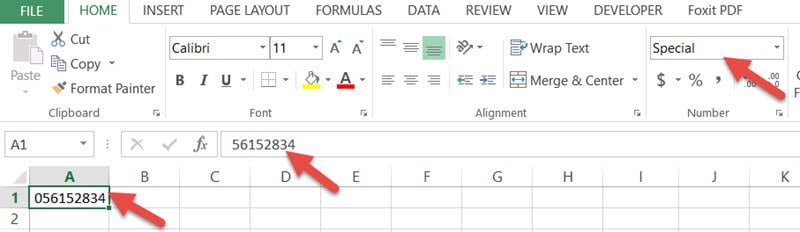In such cases, you can remove the leading zeros by just clicking the drop-down and converting the format back to General.

## Removing Leading Zeros if Cells are of Text Format

If the cells have a small green triangle on the top left corner, it means the cell was converted to text.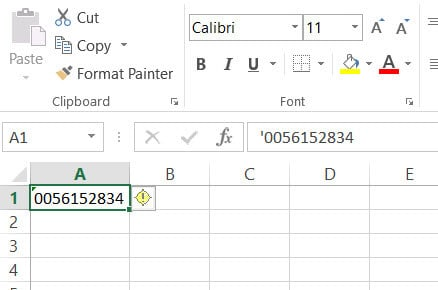If that’s the case, you will also notice the cell’s contents aligned to the left rather than to the right. Excel always aligns numbers to the right and text to left by default.

Most of the time if this happens, there’s a yellow exclamation point next to the cell. When you hover your mouse pointer over the exclamation point, you’ll see a tooltip that says “The number in this cell is formatted as text or preceded by an apostrophe”.

There are two ways to deal with this:

• Remove the leading apostrophe from the cell’s value at the formula bar, or
• Click the yellow exclamation point and select “Convert to Number” from the dropdown.

Some people use formulas that result in a text value with leading zeros. For example, a formula like =TEXT(A1, “00000000000”) will convert any number to text and add leading zeros to it if the number of digits in the original number is less than 10.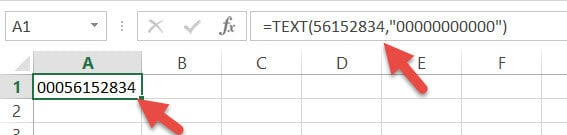If that’s the case, then you can use the VALUE() function to convert the result of the formula back to Number, thereby getting rid of the extra zeros.

So, in the above case, you can type =VALUE(A1)in cell B2.

If you want to remove the leading zeros, but also want to retain the text format, then you can use a combination of the TEXT() and VALUE() functions, like this:

 =TEXT(VALUE(B2),”#”)

The second parameter, ‘#’ means we want the format of the text not to include any extra zeros.

## A Foolproof Way to Remove Leading Zeros

If you tried out all the above methods and could still not diagnose the cause of the leading zeros, here’s a foolproof way that will work, irrespective of how the leading zeros came.

Suppose you have the following column with numbers with leading zeros and you want to remove these: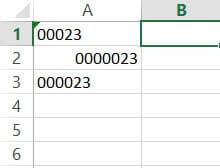Below are the steps to remove these leading zeros:

1. Create a new column.
2. Type 1 in the first cell of the column (cell B1).
3. Double click the fill handle at the bottom-right corner of the cell. This will copy the number 1 to the rest of the cells in the column.
4. Click on cell C1 and type the formula =A1*B1.
5. Now pull the fill handle to copy the formula to the rest of the cells in the column.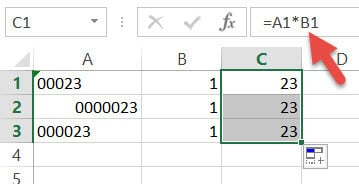Here, all you are doing is multiplying each cell value by 1. Notice that each of the cells of Column A had leading zeros put in different ways.

Some by formula, some by custom number format and some by a leading apostrophe.

## Removing a Fixed Number of Leading Zeros from a Number or Text

If you want to remove only a fixed number of zeros, instead of all the leading zeros, you can make use of a formula. This formula will work only if your original value is in Text format.

If you want to remove just one leading zero from cell A1, then use the formula:

`=IF(LEFT(A1,1) = "0", RIGHT(A1, LEN(A1)-1), A1)`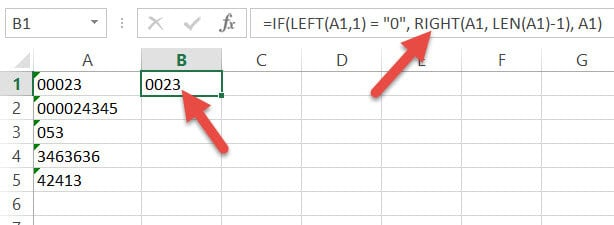What the formula does is check if the first number on the left of the value in A1 is 0. If so, then it keeps one digit less from the total length of the value, starting from its right. Otherwise, it keeps the original value as is.

If you want to remove two leading zeros from cell A1, then just drag the formula right by one cell. This will further reduce the number of zeros 1.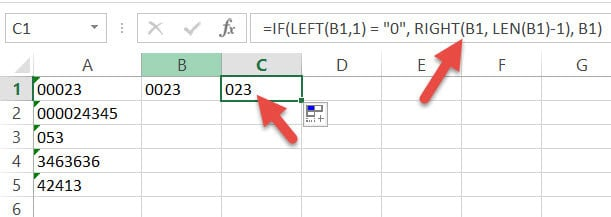Similarly, to remove three zeros, drag the formula right by one cell again.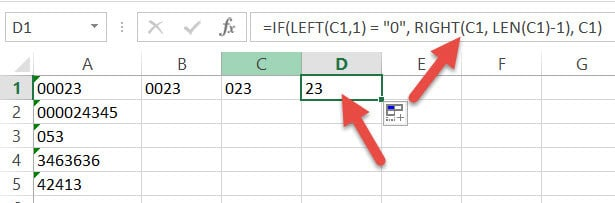Repeat this for the number of zeros you want to remove. After that, drag down the fill handle to copy the formula for all the cells in the column.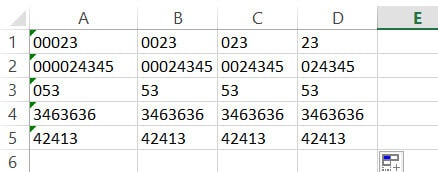Select these cells and copy them. Then, paste them as the value in the next column. Now you can delete all the intermediate columns you had created.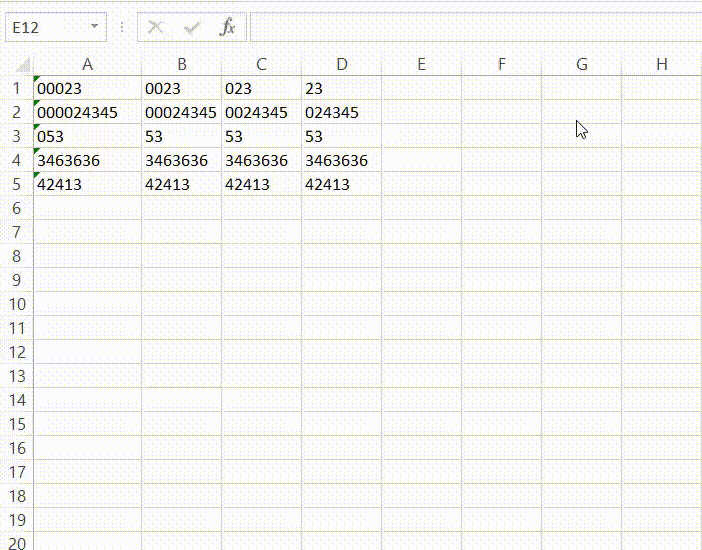## Conclusion

In this tutorial, we showed you how you can find out what caused leading zeros to appear in your cells and how you can remove them.

As a bonus, we also showed you what to do if you want to remove a fixed number of zeros, instead of all.

We hope you find the methods shown in this tutorial helpful in solving your problem.

Other Excel tutorials you may find useful: